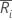# R chart

Select the method or formula of your choice.

## Plotted points

Each plotted point, ri, represents the range for subgroup i.

## Center line

The value of the center line for each subgroup,, is calculated as follows:

### Notation

TermDescription
ni number of observations in subgroup i
d2(·)value of unbiasing constant d2 that corresponds to the value specified in parentheses.
σprocess standard deviation

## Control limits

### Lower control limit (LCL)

The value of the lower control limit for each subgroupis equal to the greater of the following:

or

### Upper control limit (UCL)

The value of the upper control limit for each subgroupis calculated as follows:

### Notation

TermDescription
d2(·)value of unbiasing constant d2 that corresponds to the value specified in parentheses.
ninumber of observations in subgroup i
σprocess standard deviation
kparameter for Test 1 (The default is 3.)
d3(·)value of unbiasing constant d3 that corresponds to the value specified in parentheses.
By using this site you agree to the use of cookies for analytics and personalized content.  Read our policy